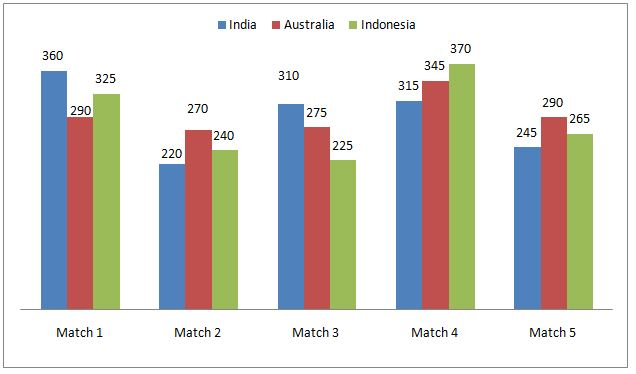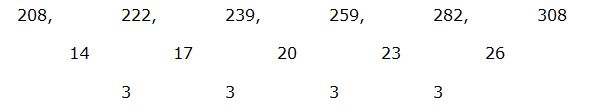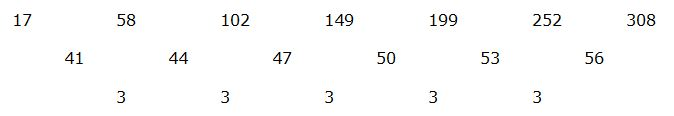# SBI PO Prelims Quantitative Aptitude Questions 2019 – Day 21

SBI PO 2019 Notification will be expected soon. It is one of the most expected recruitment among the banking aspirants. Every year the exam pattern for SBI PO has been changing. Depends upon the changing of exam pattern the questions are quite harder compare to the previous year. So the questions are in high level than the candidate’s assumption.

As per the latest trend, our IBPS Guide is providing the updated New Exam Pattern Quantitative Aptitude questions for SBI PO 2019 Day 21. Our Skilled experts were mounting the questions based on the aspirant’s needs. So candidates shall start your preparation and practice on daily basis with our SBI PO pattern quantitative aptitude questions 2019 day 21. Start your effective preparation from the right beginning to get success in upcoming SBI PO 2019.

[WpProQuiz 5402]

### Click Here for SBI PO Pre 2019 High-Quality Mocks Exactly on SBI Standard

Directions (1 – 5): The questions below are based on the given Series-I. The series-I satisfy a certain pattern, follow the same pattern in Series-II and answer the questions given below.

1)

I) 4, 3, 5, 14, 64, 528

II) 12 ……. 784. If 784 is nth term, then find the value of n?

a) 5th term

b) 4th term

c) 6th term

d) 7th term

e) None of these

2)

I) 132, 66, 22, 5.5, 1.1

II) 270 ……. 2.25. If 2.25 is nth term, then what value should come in place of

(n – 1)th term?

a) 25

b) 13.5

c) 15

d) 17.75

e) 16.5

3)

I) 208, 222, 239, 259, 282, 308

II) 17, 58 ……. 308. If 308 is (n + 2)th term, then what value should come in place of nth term?

a) 217

b) 185

c) 178

d) 199

e) 223

4)

I) 11, 15, 39, 133, 557, 2821

II) 23 …….. 4261. If 4261 is nth term, then find the value of n?

a) 5th term

b) 4th term

c) 6th term

d) 7th term

e) None of these

5)

I) 389, 391, 403, 439, 519, 669

II) 123 …… 655. If 655 is nth term, then find the value of n?

a) 7th term

b) 5th term

c) 8th term

d) 6th term

e) None of these

Directions (Q. 6 – 10): Study the following information carefully and answer the given questions:

The following line graph shows the runs scored by three different teams in 5 different cricket matches.6) The total runs scored by India and Indonesia in Match 1 and 3 together is approximately what percentage less than the total runs scored by Australia in all the given matches together?

a) 24 %

b) 32 %

c) 17 %

d) 5 %

e) 45 %

7) Find the difference between the total runs scored by India to that of Australia in all the given matches together?

a) 28 runs

b) 20 runs

c) 36 runs

d) 44 runs

e) None of these

8) Find the ratio between the total runs scored by Australia in match 1, match 3 and match 5 together to that of total runs scored by Indonesia in match 2, match 4 and match 5 together?

a) 171: 175

b) 142: 123

c) 124: 117

d) 136: 105

e) None of these

9) Which team has highest scores in the all the given matches together?

a) India

b) Indonesia

c) India & Australia

d) Australia

e) None of these

10) Total runs scored by India in match 2, match 4 together is approximately what percentage of total runs scored by Australia in match 1 and match 5 together?

a) 83 %

b) 77 %

c) 92 %

d) 105 %

e) 118 %

Directions (1-5):

Series I pattern:

4 is the first term

4*0.5 + 1 = 3

3*1 + 2 = 5

5*2 + 4 = 14

14*4 + 8 = 64

64*8 + 16 = 528

528 is 6th term

Series II pattern:

12 is the first term

12*0.5 + 1 = 7

7*1 + 2 = 9

9*2 + 4 = 22

22*4 + 8 = 96

96*8 + 16 = 784

784 is 6th term

Series I pattern:

132 is the first term

132 ÷ 2 = 66

66 ÷ 3 = 22

22 ÷ 4 = 5.5

5.5 ÷ 5 = 1.1

1.1 is 5th term

Series II pattern:

270 is the first term

270 ÷ 2 = 135

135 ÷ 3 = 45

45 ÷ 4 = 11.25

11.25 ÷ 5 = 2.25

2.25 is 5th term. (5 – 1)th term = 11.25

Series I pattern:

208 is first term. 308 is 6th term.The difference of difference is, 3, 3, 3,….

Series II pattern:

17 is first term. 308 is 7th term.308 is 7th term.

Here 308 is (n + 2)th term. So nth term is 5th term, i.e., 199

Series I pattern:

11 is the first term.

11*1 + 22 = 15

15*2 + 32 = 39

39*3 + 42 = 133

133*4 + 52 = 557

557*5 + 62 = 2821

2821 is 6th term.

Series II pattern:

23 is the first term

23*1 + 22 = 27

27*2 + 32 = 63

63*3 + 42 = 205

205*4 + 52 = 845

845*5 + 62 = 4261

4261 is 6th term

Series I pattern:

389 is first term

389 + (12 + 13) = 391

391 + (22 + 23) = 403

403 + (32 + 33) = 439

439 + (42 + 43) = 519

519 + (52 + 53) = 669

669 is 6th term

Series II pattern:

123 is first term

123 + (12 + 13) = 125

125 + (22 + 23) = 137

137 + (32 + 33) = 173

173 + (42 + 43) = 253

253 + (52 + 53) = 403

403 + (62 + 63) = 655

655 is 7th term

Directions (6-10):

The total runs scored by India and Indonesia in Match 1 and 3 together

= > 360 + 325 + 310 + 225 = 1220 runs

The total runs scored by Australia in all the given matches together

= > 290 + 270 + 275 + 345 + 290 = 1470 runs

Required % = [(1470 – 1220)/1470]*100 = 17 %

The total runs scored by India in all the given matches together

= > 360 + 220 + 310 + 315 + 245 = 1450 runs

The total runs scored by Australia in all the given matches together

= > 290 + 270 + 275 + 345 + 290 = 1470 runs

Required difference =1470 – 1450 = 20 runs

The total runs scored by Australia in match 1, match 3 and match 5 together

= > 290 + 275 + 290 = 855 runs

The total runs scored by Indonesia in match 2, match 4 and match 5 together

= > 240 + 370 + 265 = 875 runs

Required ratio = 855: 875 = 171: 175

The total runs scored by India in all the given matches together

= > 360 + 220 + 310 + 315 + 245 = 1450 runs

The total runs scored by Australia in all the given matches together

= > 290 + 270 + 275 + 345 + 290 = 1470 runs

The total runs scored by Indonesia in all the given matches together

= > 325 + 240 + 225 + 370 + 265 = 1425

Total runs scored by India in match 2 and match 4 together

= > 220 + 315 = 535

Total runs scored by Australia in match 1 and match 5 together

= > 290 + 290 = 580

Required % = (535/580)*100 = 92.24 % = 92 %